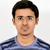0
Completed

# Use of Polyeig function

Ajay Manwani 9 years ago updated 9 years ago
Could you please provide an example of polyeig function. I would like to calculate eigen value as
A0=mp(rand(100)); A1 =mp(rand(100)); A2 =mp(randn(100))
[V,D]= polyeig(A0,A1,A2);
But this gives error
Index exceeds matrix dimensions.
Error in ==>
C:\Users\lAPi\Documents\Multiprecision
Computing Toolbox\lib\mppolyeig.p>mppolyeig
at 53

Error in ==> mp>mp.polyeig at 2691
[varargout{1:nargout}] =
mppolyeig(varargin{:});

Error in ==> trial_current_mt_highk at 255
[V,D]= polyeig(A0,A1,A2);Completed